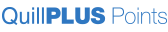Limited Time - Enroll today for only \$4.99
Close
Scientific Calculators  (27 Products)
Scientific calculators take users beyond simple addition, subtraction, multiplication, and division. They can handle algebra or trigonometry equations and can even show graphs or pie charts of the calculations. Solve difficult math problems with ease. Quill has many calculators with different functions and features to fit your math or engineering needs. Read More
Sort By: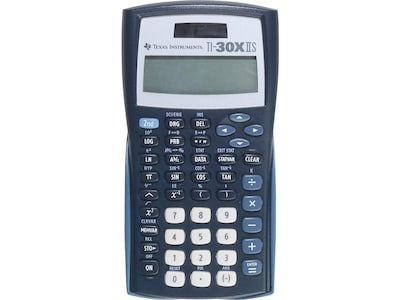### Texas Instruments TI-30XIIS 10-Digit Scientific Calculator, Blue

Scientific calculator two-line LCD display: 10-digit answer & two-digit exponent line
Perfect choice for general math, statistics, pre-algebra, general science
Calculator works from solar cell and one CR2025 battery
\$16.69 Each
Item # 901-TI30XII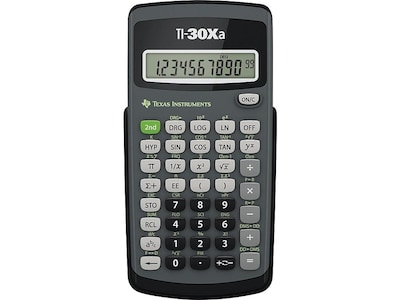### Texas Instruments TI-30Xa 10-Digit Scientific Calculator, Black

Scientific calculator with 10-digit one-line LCD display
Perfect choice for general math, algebra 1 and 2, pre-algebra, general science
Calculator works from one LR44 battery
\$11.59 Each
Item # 901-TI30XA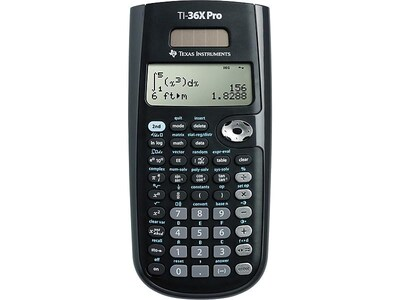### Texas Instruments TI-36X Pro 16-Digit Scientific Calculator, Black

Scientific calculator with 16-digit four-line LCD display
Perfect choice for geometry, trigonometry, statistics, biology, chemistry, physics, algebra 1 and 2
Calculator works from solar cell and one CR2032 battery
\$22.99 Each
Item # 901-321966
Guaranteed 1-2 Day Delivery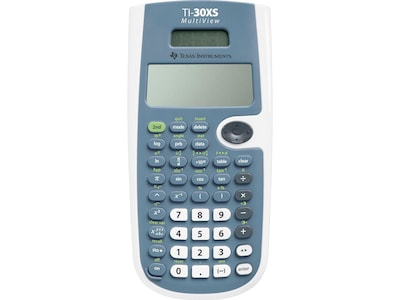### Texas Instruments MultiView TI-30XS 16 Digit Scientific Calculator, Blue/White

Scientific calculator with 16-digit four-line LCD display
Perfect choice for general math, statistics, pre-algebra, general science, geometry, chemistry
Calculator works from solar cell and one battery
\$17.69 Each
Item # 901-TI30XS
Guaranteed 1-2 Day Delivery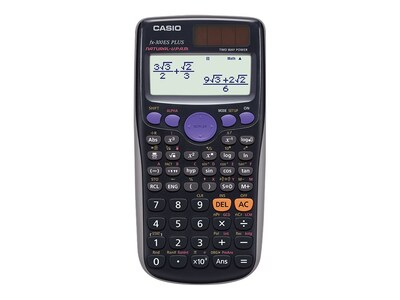### Casio FX-300ES Plus 16-Digit Scientific Calculator, Black

Powerful scientific calculator with 16-digit four-line LCD display
Calculator for general math, trigonometry, statistics, algebra, geometry, and physics
Dimensions: 0.38"H x 3.13"W x 6.38"D
\$15.59 Each
Item # 901-FX300ES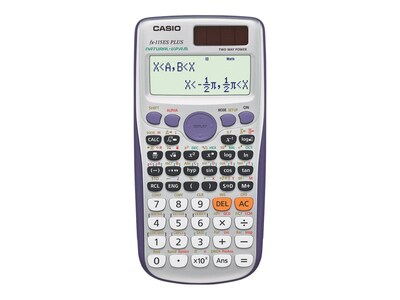### Casio FX-115ES Plus 16-Digit Scientific Calculator, Gray

Powerful scientific calculator with 16-digit four-line LCD display
Perfect choice for general math, trigonometry, statistics, algebra, engineering, and physics
Dimensions: 0.38"H x 3.13"W x 6.38"D
\$21.99 Each
Item # 901-FX115ESPLUS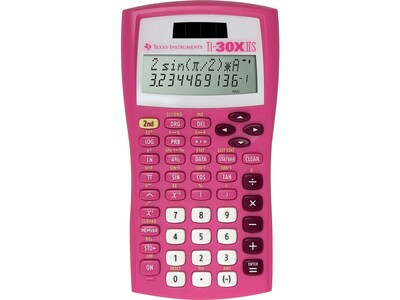### Texas Instruments TI-30XIIS 10-Digit Scientific Calculator, Pink

Scientific calculator two-line LCD display: 10-digit answer & two-digit exponent line
Perfect choice for general math, statistics, pre-algebra, general science
Calculator works from solar cell and one CR2025 battery
\$16.79 Each
Item # 901-862871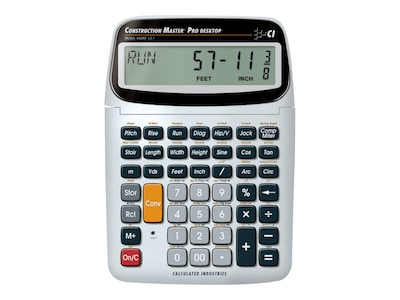### Calculated Industries Construction Master 44080 11-digit Construction Calculator, Silver/Black

Construction calculator with 11 digits LCD
Perfect for all construction challenges: saves time, reduces costs, and prevents rework
Dimensions: 9"H x 6.4"W x 1.4"D
\$102.99 Each
Item # 901-1199929CAL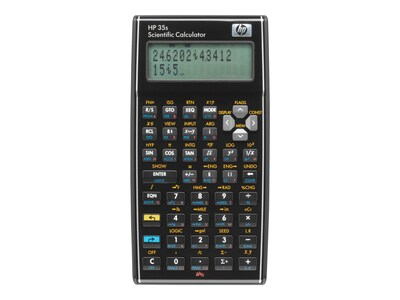### HP 35s 14-Digit Scientific Calculator, Black

Scientific calculator with 14-digit two-line LCD display
Ideal choice for engineering, surveying, science, and statistics
Dimensions: 6.22"H x 3.23"W x 0.72"D
\$63.99 Each
Item # 901-919140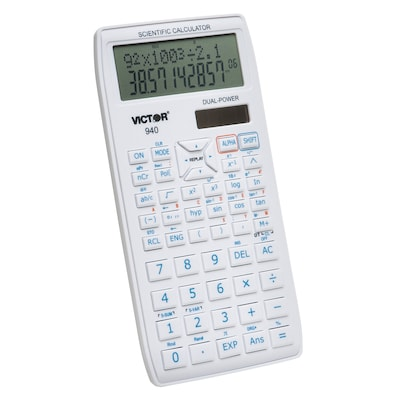### Victor Technology Scientific Calculator with 2 Line Display, VCT940, 10 digit

White, 2 line display, 10 digit
General Math, Algebra 1 & 2, Science, Statistics, Trigonometry, and Geometry
Quadratic and cubic equations, coordinate conversion, trigonometric functions in degrees and radians, fractions, reciprocals, factorials, basic scientific functions
\$8.99 Each
Item # 901-JV66698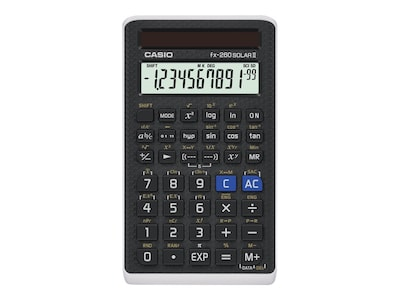### Casio FX-260 Solar II 10-Digit Scientific Calculator, Black

Powerful scientific calculator with 10-digit LCD display
All purpose scientific calculator offers fraction calculations, trigonometric functions and more
Dimensions: 0.38"H x 2.75"W x 4.75"D
\$10.39 Each
Item # 901-2657917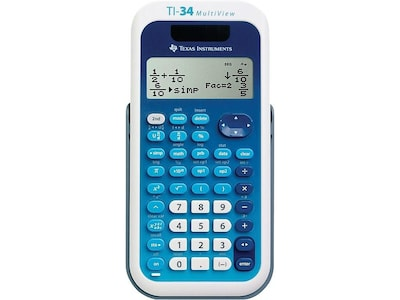### Texas Instruments MultiView TI-34 16 Digit Scientific Calculator, Blue/White

Scientific calculator with 16-digit four-line LCD display
Perfect choice for middle school math, pre-algebra, algebra 1 and 2, general science, geometry
Calculator works from solar cell and one (CR2032) battery
\$22.59 Each
Item # 901-733108TEX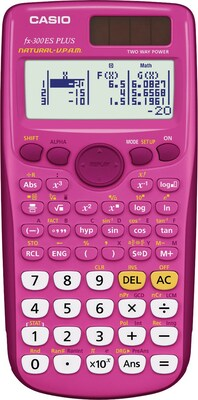### Casio FX-300ES PLUS Scientific Calculator, Pink

Over 250 built-in math functions including: basic and advanced scientific, exponential and trigonometric, fractions, regression analysis, and more
Mult-replay function lets user step back through calculations, edit and recalculate
1 independent memory and 6 constant memories
\$15.29 Each
Item # 901-754468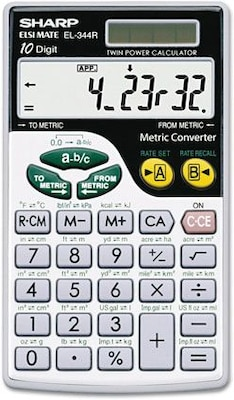### Wallet Calculator, Extra-Large Display, 10 Digit Screen, Metric Conversion, Solar Power, 2-3/4"x4-4/5"

Extra-large display
2.75"Wx4.875"D
Pocket Solar Calculator with Metric Converter
\$28.99 Each
Item # 901-EL344RB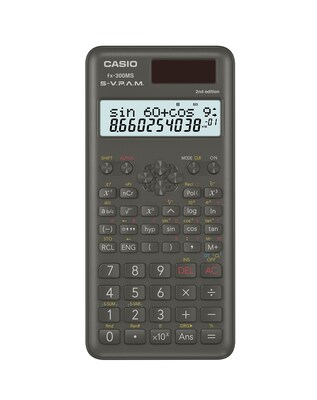### Casio FX 300MSPLUS2 12 Digit 2-Line Display Scientific Calculator, Black

Fraction Calculations
Multi-Replay Function
Trigonometric / Inverse Trigonometric Functions
\$10.99 Each
Item # 901-24387203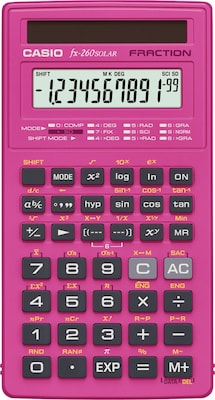### Casio FX260SLRS Pink Solar Scientific Calculator, 144 built-in functions

All-purpose scientific calculator, offers fraction calculation and trigonometric functions
Power source: Solar
Display notation: Numeric, display type: LCD
\$9.89 Each
Item # 901-2716153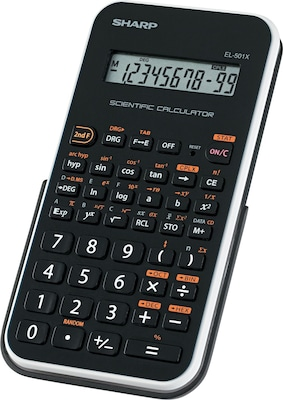### Sharp® EL-501XBWH Scientific Calculator, 10-Digit LCD, Black/White

Ideal for students studying general math, pre-algebra, algebra, geometry and trigonometry
Performs more than 130 scientific and math functions
Hard cover protects calculator from scratches
\$8.79 Each
Item # 901-EL501XBWH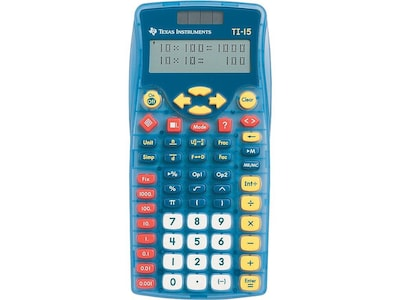### Texas Instruments Explorer TI-15 11-Digit Scientific Calculator, Blue

Scientific Calculator with 11-digit two-line display
Help to prepare your child or students for their next steps in math and science
Calculator works from solar cell and battery
\$20.59 Each
Item # 901-TI15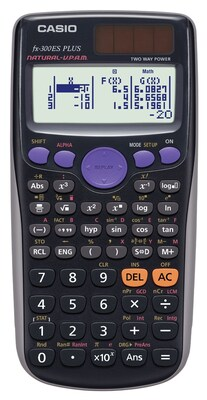### Casio® FX300ESPLS Teacher Pack Engineering/Scientific Calculator, 10/Pack

Black
Input voltage: 115 - 230 VAC
Output voltage: +3.3 V at 25 A, +5 V at 25 A, +12 V1 at 60 A, +12 V2 at 80 A, -12 V at 0.3 A, +5 VSB at 3.5 A
\$138.99 Each
Item # 901-895861DHT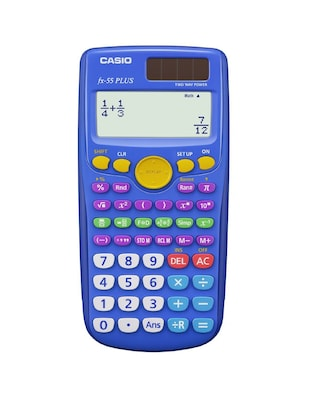### Casio® FX-55PLUS Fraction Scientific Calculator Teacher Pack, 10/Pack

Display type: Dot Matrix
Display screen: 12 digit - Dot Matrix
Power source: Battery
\$143.99 Each
Item # 901-895862DHT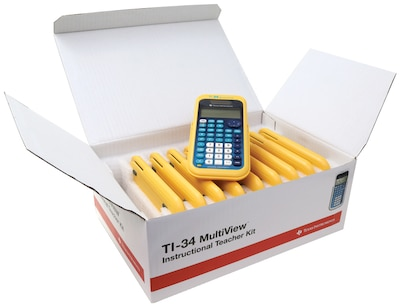### Texas Instruments Multiview TI-34 16-Digit Scientific Calculator, Yellow/Blue, Teacher 10 Pack

Scientific calculator with 16-digit four-line LCD display
Perfect choice for middle school math, pre-algebra, algebra 1 and 2, general science, geometry
Calculator works from solar cell and one (CR2032) battery
\$180.99 Each
Item # 901-904451DHT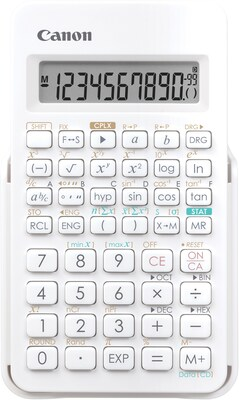### Canon F-605 Scientific Calculator

Great for Algebra 1& 2, Biology computing, General Math, Geometry, pre-algebra, statistics and trigonomtery
Total 154 Functions including Memory, Fraction, Trigonometry, Complex Number, Base-N, Statistics
10+2 digits
\$10.79 Each
Item # 901-2145183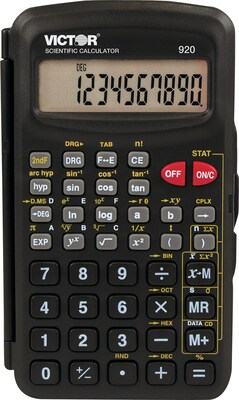### 920 Compact Scientific Calculator With Hinged Case,10-Digit, LCD

Ten-digit scientific calculator is perfect for students in General Math, Science, Algebra and Trigonometry
This calculator features hex/dec/oct/bin and decimal degrees conversions
Other functions include: logarithms, reciprocals, powers, roots, factorials, trigs, hyperbolic trigs and one variable statistical calculation
\$8.79 Each
Item # 901-2681640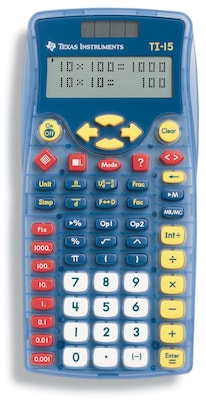### Texas Instruments TI15TK 11 Digit Scientific Calculator, Blue

No. of digits: 11-digit display
Display type: LCD
Display screen: 2 line x 11-digit
\$169.99 Each
Item # 901-904473DHT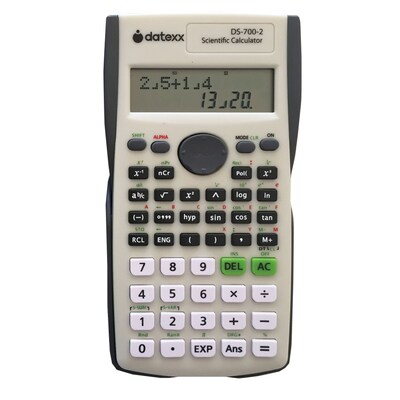### Datexx DS-7002-10 2-Line Scientific Calculator, White 10/Pack

Two line alphanumeric display
Over 200 functions
Solar and battery powered
\$134.99 Pack
Item # 901-DS700210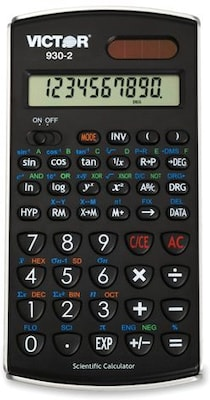### Victor 930-2 10 Digit Scientific Calculator with Solar Power

10-digit, 2 digit exponent
Protective hard shell case
Performs 154 functions, which make this model perfect for students in General Math, Science, Algebra and Trigonometry. Large, easy to read LCD display with two-digit exponent
\$16.49 Each
Item # 901-919335### Datexx 2 Line Scientific Calculator

Easy-to-Read, 2-Line Alphanumeric 10+2 Digit Display
224 Functions
9 Memories
\$14.99 Each
Item # 901-24316194
SS,121242
Want to know more about Scientific Calculators

## Number of functions of scientific calculators

Most calculators with scientific abilities perform a minimum of 100 different functions. These functions include math performed on simple calculators as well as trigonometry, exponents, statistic equations, and algebra. Some calculators can perform over 500 functions, including the ability to calculate binary, decimal, octal, and hexadecimal numbers. It is even possible to enter formulas with letters into the calculator.

## Digits and lines on scientific Casio calculators

In order to calculate so many formulas and problems, scientific calculators need the space to do so. Most calculators include the ability to type one line of numbers of 8 to 10 digits. The long line allows for large, high number calculations. Some calculators have up to four lines of 8 to 10 numbers. Users can have rows of number calculations in order to see how the calculation would look if on paper. Lines also allow for more tricky formulas.

## The added function of graphing calculators

Graphing calculators are a type of scientific calculator that also shows graphs of the calculations on the screen. Users can create line graphs, bar charts, and even pie charts that help to visualize the math. Since graphs require ample space, most graphing calculators have several lines on the screen for other calculations.

## Printing calculators for financial calculations

While most scientific calculators are designed for science and engineering there are some that help with business. All printing calculators have a built-in printer with a calculator paper roll that prints out the calculations. They contain several financial functions including sales tax to make business sales easier. These calculators are useful for businesses and accountants that want instant access to printed information.

## Other features found with scientific calculators

It might seem that these calculators do enough already but the above functions are just the beginning. Here are some other features found with many calculators:

• Solar powered
• Display math formulas and equations as written in textbooks
• Save calculations in multiple memory banks
• USB port to transfer calculations to computer
• Programmable keys for custom functions
• Built-in libraries with unit conversions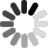Loading...